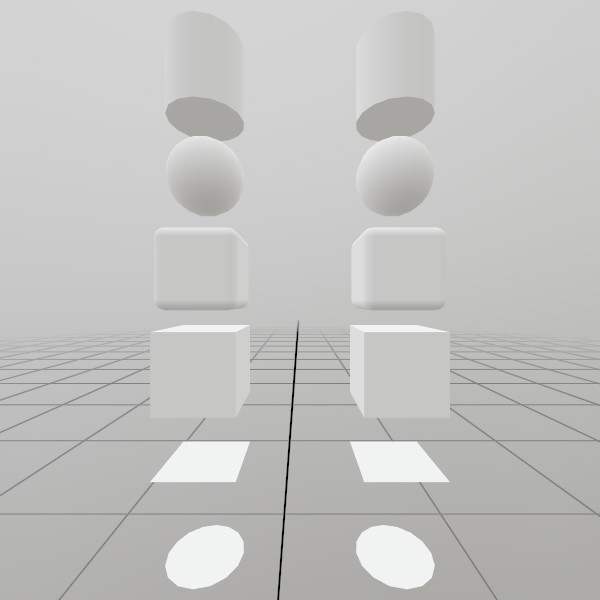# Mesh.GeneratePlane

static Mesh GeneratePlane(Vec2 dimensions, int subdivisions)

Vec2 dimensions How large is this plane on the XZ axis, in meters?
int subdivisions Use this to add extra slices of vertices across the plane. This can be useful for some types of vertex-based effects!
RETURNS: Mesh A plane mesh, pre-sized to the given dimensions.

Generates a plane on the XZ axis facing up that is optionally subdivided, pre-sized to the given dimensions. UV coordinates start at 0,0 at the -X,-Z corer, and go to 1,1 at the +X,+Z corner!

static Mesh GeneratePlane(Vec2 dimensions, Vec3 planeNormal, Vec3 planeTopDirection, int subdivisions)

Vec2 dimensions How large is this plane on the XZ axis, in meters?
Vec3 planeNormal What is the normal of the surface this plane is generated on?
Vec3 planeTopDirection A normal defines the plane, but this is technically a rectangle on the plane. So which direction is up? It’s important for UVs, but doesn’t need to be exact. This function takes the planeNormal as law, and uses this vector to find the right and up vectors via cross-products.
int subdivisions Use this to add extra slices of vertices across the plane. This can be useful for some types of vertex-based effects!
RETURNS: Mesh A plane mesh, pre-sized to the given dimensions.

Generates a plane with an arbitrary orientation that is optionally subdivided, pre-sized to the given dimensions. UV coordinates start at the top left indicated with ‘planeTopDirection’.

## ExamplesHere’s a quick example of generating a mesh! You can store it in just a Mesh, or you can attach it to a Model for easier rendering later on.

``````Mesh  planeMesh  = Mesh.GeneratePlane(Vec2.One*0.4f);
Model planeModel = Model.FromMesh(planeMesh, Default.Material);
``````

Drawing both a Mesh and a Model generated this way is reasonably simple, here’s a short example! For the Mesh, you’ll need to create your own material, we just loaded up the default Material here.

``````Matrix planeTransform = Matrix.T(1.0f, 0, 1);